# PHP内核探索之变量（3）- hash table

+关注继续查看

在PHP中，除了zval, 另一个比较重要的数据结构非hash table莫属，例如我们最常见的数组，在底层便是hash table。除了数组，在线程安全（TSRM）、GC、资源管理、Global变量、ini配置管理中，几乎都有Hash table的踪迹（上一次我们也提到，符号表也是使用Hash table实现的）。那么，在PHP中，这种数据有什么特殊之处，结构是怎么实现的？ 带着这些问题，我们开始本次的内核探索之旅。

本文主要内容：

1. Hash table的基本介绍
2. PHP底层Hash table的结构和实现
3. Zend Hash table API

### 一、Hash table的基本介绍和背景知识

1.    基本定义

Hash table,又叫哈希表，散列表，Hash表，维基百科上对哈希表的定义是："散列表，是根据关键字（Key value）而直接访问在内存存储位置的数据结构。也就是说，它通过把键值通过一个函数的计算，映射到表中一个位置来访问记录，这加快了查找速度。这个映射函数称做散列函数，存放记录的数组称做散列表。”。提取文中的主干，我们可以得出如下信息：

（1）.Hash table是一种数据结构。

（2）.这种数据结构是普通数组的扩展。

（3）.这种数据结构通过key->value的映射关系，使得插入和查找的效率很高（数组可以直接寻址，可在O(1)的时间内访问任意元素）。

我们知道，在一般的数组、线性表、树中，记录在结构中的位置是相对随机的，即记录和关键字之间不存在直接的、确定的对应关系。在这些结构中，要查找和插入关键字，常常需要进行一系列的比较，查找的效率通常是O(n)或者O(lgn)的。而Hash table通过Hash函数建立了关键字和记录之间的对应关系，使得普通的查找和插入操作可以在O(1)（平均时间复杂度）的时间内完成，这显然是最理想的查找方式。

2.    Hash函数

如上所述，Hash函数建立了关键字和记录之间的对应关系,即：Record = Hash(key) , 这种对应关系如下所示：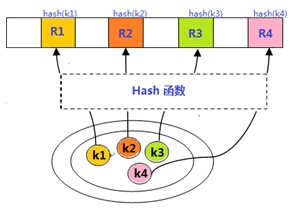function hash( $key ){$result = 0;
$len = strlen($key);

for($i = 0;$i < $len;$i++ ){
$result += ord($key{$i}) * ((1 << 5) + 1); } return$result;
}


3.冲突解决

在理想的情况下，我们期望任何关键字计算出的Hash值都是唯一的，这样我们便可以通过Hash(key)这种方式直接定位到要查找的记录。但不幸的，几乎没有一个Hash函数可以满足这样的特性（即使有这样的Hash函数，也可能很复杂，无法在实际中使用）。也就是说，即使是精心设计的Hash函数，也经常会出现key1 != key2 但是hash(key1) = hash(key2)的情况，这便是Hash冲突（Hash碰撞）。解决Hash碰撞的主要方法有多种（见这里），作为示例，我们只简单讨论下链接法解决冲突。这种方法的基本思想是：在哈希表出现冲突时，使用链表的形式链接所有具有相同hash值的记录，而哈希表中只保存链表的头指针。PHP底层的Hash table，便是使用链表（双向链表）来解决hash冲突的。关于这一点，后续会有详细的介绍。

引入链表之后，Hash table的结构如下所示：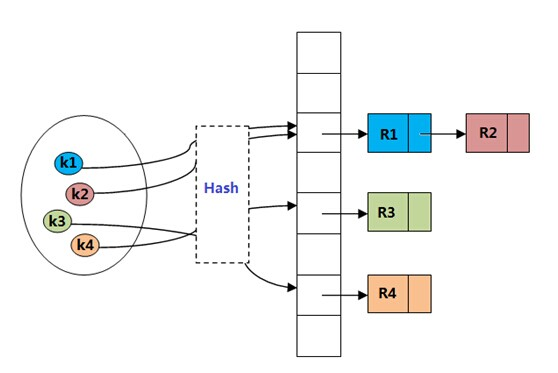一个简单的Hash table的实现如下：

Class HashTable{
private $buckets = null; /* current size */ private$size = 0;

/* max hashtable size */
private $max = 2048; private$mask = 0;

public function __construct($size){$this->_init_hash($size); } /* hashtable init */ private function _init_hash($size){
if($size >$this->max){
$size =$this->max;
}

$this->size =$size;
$this->mask =$this->size - 1;

// SplFixedArray is faster when the size is known
// see http://php.net/manual/en/class.splfixedarray.php
$this->buckets = new SplFixedArray($this->size);
}

public function hash( $key ){$result = 0;
$len = strlen($key);

for($i = 0;$i < $len;$i++ ){
$result += ord($key{$i}) * ((1 << 5) + 1); } return$result % ($this->size); } /* 拉链法 */ public function insert($key, $val ){$h = $this->hash($key);
if(!isset($this->buckets[$h])){
$next = NULL; }else{$next = $this->bucket[$h];
}
$this->buckets[$h] = new Node($key,$val, $next); } /* 拉链法 */ public function lookup($key ){
$h =$this->hash($key);$cur = $this->buckets[$h];

while($cur !== NULL){ if($cur->key == $key){ return$cur->value;
}
$cur =$cur->next;
}
return NULL;
}
}

Class Node{
public $key; public$value;
public $next = null; public function __construct($key, $value,$next = null){
$this->key =$key;
$this->value =$value;
$this->next =$next;
}
}

$hash = new HashTable(200);$hash->insert('apple','this is apple');
$hash->insert('orange','this is orange');$hash->insert('banana','this is banana');
echo $hash->lookup('apple');  我们知道，在PHP中，数组支持k->v这样的关联数组，也支持普通的数组。不仅支持直接寻址（根据关键字直接定位），而且支持线性遍历（foreach等）。这都要归功于Hash table这一强大和灵活的数据结构。那么，在PHP底层，Hash table究竟是如何实现的呢？我们一步步来看。 ### 二、PHP中Hash table的基本结构和实现 1. 基本数据结构 在PHP底层，与Hash table相关的结构定义、算法实现都位于Zend/zend_hash.c和Zend/zend_hash.h这两个文件中。PHP 的hash table实现包括两个重要的数据结构，一个是HashTable,另一个是bucket.前者是hash table的主体，后者则是构成链表的每个“结点”,是真正数据存储的容器。 （1） HashTable的基本结构 定义如下（zend_hash.h）： typedef struct _hashtable { uint nTableSize; uint nTableMask; uint nNumOfElements; ulong nNextFreeElement; Bucket *pInternalPointer; /* Used for element traversal */ Bucket *pListHead; Bucket *pListTail; Bucket **arBuckets; dtor_func_t pDestructor; zend_bool persistent; unsigned char nApplyCount; zend_bool bApplyProtection; #if ZEND_DEBUG int inconsistent; #endif } HashTable; 这是一个结构体，其中比较重要的几个成员： nTableSize 这个成员用于标明Hash表的大小，在hash表初始化操作的时候，会设定nTableSize的大小，而在hash表扩容的时候，也会相应调整这个数值的大小。注意这个数值并不是hash表中元素的个数。 nTableMask 是一个“掩码”，主要用于快速计算一个元素的索引（nIndex = h & ht->nTableMask，在一般的Hash函数中，是通过模运算来确定索引的，但显然，位运算比模运算效率要高），在arBuckets初始化之后，该值默认固定为nTableSize – 1; nNumOfElements 这个成员保存了hashtable中保存的元素的个数，通常情况下，我们在PHP脚本中使用count($arr)与这个结果是一致的（参见ext/standard/array.c）

nNextFreeElement  这个字段记录下一个可用的索引位置，我们在脚本中使用$array[] = 'key'的时候，就是使用nNextFreeElement给出的索引值（zend_hash.c）： if (flag & HASH_NEXT_INSERT) { h = ht->nNextFreeElement; } pInternalPointer 这是一个指针。在PHP脚本中，我们使用current,next,key,end等 与数组相关的操作时，都是使用pInternalPointer这一指针来完成的。 pListHeadpListTail PHP底层实际上维护了两个重要的数据结构，除了hash表（以及用于解决冲突的双链表），还有一个双向链表用于hash表元素的线性扫描。pListHead和pListTail便指向这个双链表的表头和表尾。 arBuckets 这是一个bucket *类型的数组，数组中每个元素都是一个bucket* 的指针，具有相同hash值的元素通过bucket的pNext和pLast指针连接成一个双链表（这个双链表与前面说的用于线性遍历的双链表并不是一个东西）。因此，bucket是实际存储数据的容器。 nApplyCountbApplyProtection 提供了一种保护机制，主要是用于防止循环引用导致的无限递归。 persistent 这是一个布尔变量，该变量会影响到内存分配的方式，这涉及到PHP内存管理的一些知识，我们暂时不做更多解释，详细的可以参考： http://cn2.php.net/manual/en/internals2.memory.persistence.php （2）另一个数据结构是Bucket 该结构的定义为： typedef struct bucket { ulong h; uint nKeyLength; void *pData; void *pDataPtr; struct bucket *pListNext; struct bucket *pListLast; struct bucket *pNext; struct bucket *pLast; const char *arKey; } Bucket; 其中： h ,arKey,nKeyLength PHP数组中，有两类不同的索引，一类是数字索引，这与C中的数组非常类似(如$arr = array(1=>'cont')), 另一类是字符串索引，也就是使用关键词作为数组项的索引（如$arr = array('index'=>'cont');）.这两类索引在PHP底层是通过不同的机制来区分的：对于数字型索引，直接使用h作为hash值，同时，arKey=NULL 且nKeyLength=0, 而对于字符串索引，arKey保存字符串key, nKeyLength保存该key的长度，h则是该字符串通过hash函数计算后的hash值。这样，在PHP中，实际上通过h, arKey, nKeyLength来唯一确定数组中的一个元素的，这从zend_hash_key这个结构体的定义也可以看出来： typedef struct _zend_hash_key { const char *arKey; uint nKeyLength; ulong h; } zend_hash_key; 而确定数组元素在hashtable中的位置则是通过h & ht->nTableMask 来实现的： /* 字符串型索引 */ h = zend_inline_hash_func(arKey, nKeyLength); nIndex = h & ht->nTableMask; /* 数字型索引-append$arr[] = 'test';这种形式 */
if (flag & HASH_NEXT_INSERT) {
h = ht->nNextFreeElement;
}

/* 指定数字索引时直接使用h */
nIndex = h & ht->nTableMask;


pDatapDataPtr  通常情况下，Bucket中的数据是保存在pData指针指向的内存空间的。但是也有例外，例如保存的是一个指针。这时，pDataPtr指向该指针，而pData指向pDataPtr。这从INIT_DATA这个宏定义可以看出来：

#define INIT_DATA(ht, p, pData, nDataSize);                             \
if (nDataSize == sizeof(void*)) {                                   \
memcpy(&(p)->pDataPtr, pData, sizeof(void *));                  \
(p)->pData=&(p)->pDataPtr;                                      \
}else{                                                              \
(p)->pData = (void *) pemalloc_rel(nDataSize, (ht)->persistent);\
if(!(p)->pData){                                                \
pefree_rel(p, (ht)->persistent);                            \
return FAILURE;                                             \
}                                                               \
memcpy((p)->pData,pData,nDataSize);                             \
(p)->pDataPtr=NULL;                                             \
}


pListNextpListLastpNextpLast 前面已经介绍过,pListNext和pListLast构成了用于遍历的整个双链表。而pNext和pLast则是在出现hash冲突时，用于链接具有相同hash值的Bucket。这两种双链表的结构分别如下图所示：

a. 发生hash冲突时的双链表：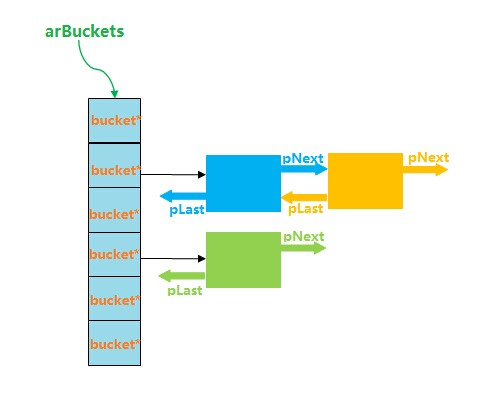b. 用于全局的双链表：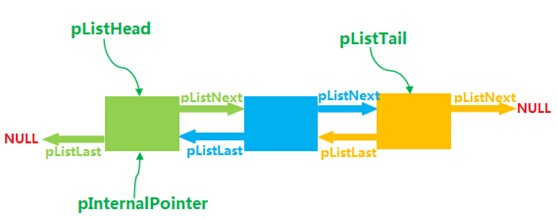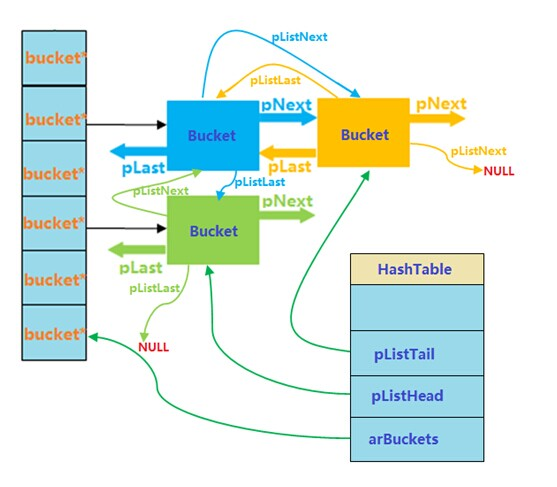### 三、HashTable的实现

1.   HashTable相关宏定义

#define CONNECT_TO_BUCKET_DLLIST(element, list_head)       \
(element)->pNext = (list_head);                         \
(element)->pLast = NULL;                                \
if ((element)->pNext) {                                 \
(element)->pNext->pLast = (element);                \
}

(2). CONNECT_TO_GLOBAL_DLLIST(element, ht)

 1 #define CONNECT_TO_GLOBAL_DLLIST(element, ht)               \
2     (element)->pListLast = (ht)->pListTail;                 \
3     (ht)->pListTail = (element);                            \
4     (element)->pListNext = NULL;                          \
5     if ((element)->pListLast != NULL) {                     \
6         (element)->pListLast->pListNext = (element);        \
7     }                                                                           \
8
9     if (!(ht)->pListHead) {                                             \
10         (ht)->pListHead = (element);                               \
11     }                                                                            \
12
13     if ((ht)->pInternalPointer == NULL) {                          \
14         (ht)->pInternalPointer = (element);                      \
15     }

(3). HASH_PROTECT_RECURSION(ht)

#define HASH_PROTECT_RECURSION(ht) \
if ((ht)->bApplyProtection) { \
if ((ht)->nApplyCount++ >= 3) { \
zend_error(E_ERROR, "Nesting level too deep - recursive dependency?");\
} \
}

(4). ZEND_HASH_IF_FULL_DO_RESIZE(ht)

HashTable的大小并不是固定不变的，当nNumOfElements > nTableSize时，会对HashTable进行扩容，以便于容纳更多的元素，这便是通过该宏实现的（实际上是调用zend_hash_do_resize来实现的）。该宏定义为：

#define ZEND_HASH_IF_FULL_DO_RESIZE(ht)             \
if ((ht)->nNumOfElements > (ht)->nTableSize) {  \
zend_hash_do_resize(ht);                    \
}

(5). INIT_DATA(ht, p, pData, nDataSize)

if (nDataSize == sizeof(void*)) {
memcpy(&(p)->pDataPtr, pData, sizeof(void *));
(p)->pData = &(p)->pDataPtr;
}


else {
(p)->pData = (void *) pemalloc_rel(nDataSize, (ht)->persistent);
if (!(p)->pData) {
pefree_rel(p, (ht)->persistent);
return FAILURE;
}
memcpy((p)->pData, pData, nDataSize);
(p)->pDataPtr=NULL;
}


#define UPDATE_DATA(ht, p, pData, nDataSize)                                            \
if (nDataSize == sizeof(void*)) {                                                   \
if ((p)->pData != &(p)->pDataPtr) {                                             \
pefree_rel((p)->pData, (ht)->persistent);                                   \
}                                                                               \
memcpy(&(p)->pDataPtr, pData, sizeof(void *));                                  \
(p)->pData = &(p)->pDataPtr;                                                    \
} else {                                                                            \
if ((p)->pData == &(p)->pDataPtr) {                                             \
(p)->pData = (void *) pemalloc_rel(nDataSize, (ht)->persistent);            \
(p)->pDataPtr=NULL;                                                         \
} else {                                                                        \
(p)->pData = (void *) perealloc_rel((p)->pData, nDataSize, (ht)->persistent);\
/* (p)->pDataPtr is already NULL so no need to initialize it */             \
}                                                                               \
memcpy((p)->pData, pData, nDataSize);                                           \
}

(6). UPDATE_DATA(ht, p, pData, nDataSize)

#define UPDATE_DATA(ht, p, pData, nDataSize)                                            \
if (nDataSize == sizeof(void*)) {                                                   \
if ((p)->pData != &(p)->pDataPtr) {                                             \
pefree_rel((p)->pData, (ht)->persistent);                                   \
}                                                                               \
memcpy(&(p)->pDataPtr, pData, sizeof(void *));                                  \
(p)->pData = &(p)->pDataPtr;                                                    \
} else {                                                                            \
if ((p)->pData == &(p)->pDataPtr) {                                             \
(p)->pData = (void *) pemalloc_rel(nDataSize, (ht)->persistent);            \
(p)->pDataPtr=NULL;                                                         \
} else {                                                                        \
(p)->pData = (void *) perealloc_rel((p)->pData, nDataSize, (ht)->persistent);   \
/* (p)->pDataPtr is already NULL so no need to initialize it */             \
}                                                                               \
memcpy((p)->pData, pData, nDataSize);                                           \
}


(7). CHECK_INIT(ht)

#define CHECK_INIT(ht) do {                                             \
if (UNEXPECTED((ht)->nTableMask == 0)) {                                \
(ht)->arBuckets = (Bucket **) pecalloc((ht)->nTableSize, sizeof(Bucket *), (ht)->persistent);   \
(ht)->nTableMask = (ht)->nTableSize - 1;                        \
}                                                                   \
} while (0)

2.   哈希函数

写这篇文章的时候，发现鸟哥已经写了一篇《PHP中的hash算法》，里边对hash的算法、思想等都做了比较详细的解答，这里就不在做过多的解释，只说一点：unrolled。unrolled本身是展开的意思，对于nKeyLength长度的key, PHP的hash算法会以8为单位做unrolled，也就是这样的形式：

for (; nKeyLength >= 8; nKeyLength -= 8) {
hash = ((hash << 5) + hash) + *arKey++;
hash = ((hash << 5) + hash) + *arKey++;
hash = ((hash << 5) + hash) + *arKey++;
hash = ((hash << 5) + hash) + *arKey++;
hash = ((hash << 5) + hash) + *arKey++;
hash = ((hash << 5) + hash) + *arKey++;
hash = ((hash << 5) + hash) + *arKey++;
hash = ((hash << 5) + hash) + *arKey++;
}


for(;nKeyLength > 0; nKeyLength--){
hash = ((hash << 5) + hash) + *arKey++;
}


/*
* 1. inline static 是为了提高效率
* 2. const限定arKey, 表明在函数中arKey的内容不应该不修改
*/

static inline ulong zend_inline_hash_func(const char *arKey, uint nKeyLength)
{
/* 3.register变量，也是为了提高效率 */
register ulong hash = 5381;

/* 4. variant with the hash unrolled eight times */
for (; nKeyLength >= 8; nKeyLength -= 8) {
hash = ((hash << 5) + hash) + *arKey++;
hash = ((hash << 5) + hash) + *arKey++;
hash = ((hash << 5) + hash) + *arKey++;
hash = ((hash << 5) + hash) + *arKey++;
hash = ((hash << 5) + hash) + *arKey++;
hash = ((hash << 5) + hash) + *arKey++;
hash = ((hash << 5) + hash) + *arKey++;
hash = ((hash << 5) + hash) + *arKey++;
}

switch (nKeyLength) {
case 7: hash = ((hash << 5) + hash) + *arKey++; /* fallthrough... */
case 6: hash = ((hash << 5) + hash) + *arKey++; /* fallthrough... */
case 5: hash = ((hash << 5) + hash) + *arKey++; /* fallthrough... */
case 4: hash = ((hash << 5) + hash) + *arKey++; /* fallthrough... */
case 3: hash = ((hash << 5) + hash) + *arKey++; /* fallthrough... */
case 2: hash = ((hash << 5) + hash) + *arKey++; /* fallthrough... */
case 1: hash = ((hash << 5) + hash) + *arKey++; break;
case 0: break;
EMPTY_SWITCH_DEFAULT_CASE()
}
/* 5. 返回的hash值并没有经过取模运算 */
return hash;
}


3.   初始化、添加/更新和查找、删除等API

(1). 初始化

ZEND_API int _zend_hash_init(HashTable *ht, uint nSize, hash_func_t pHashFunction, dtor_func_t pDestructor, zend_bool persistent ZEND_FILE_LINE_DC)
{
/* hashTable最小size为 1<<3 = 8 */
uint i = 3;

SET_INCONSISTENT(HT_OK);
if (nSize >= 0x80000000) {
/* prevent overflow */
ht->nTableSize = 0x80000000;
} else {
while ((1U << i) < nSize) {
i++;
}
ht->nTableSize = 1 << i;
}

ht->nTableMask = 0; /* 0 means that ht->arBuckets is uninitialized */
ht->pDestructor = pDestructor;
ht->arBuckets = (Bucket**)&uninitialized_bucket;
ht->pListTail = NULL;
ht->nNumOfElements = 0;
ht->nNextFreeElement = 0;
ht->pInternalPointer = NULL;
ht->persistent = persistent;
ht->nApplyCount = 0;
ht->bApplyProtection = 1;
return SUCCESS;
}


(2). 查找元素。

/* 数字型索引的查找 */
ZEND_API int zend_hash_index_find(const HashTable *ht, ulong h, void **pData)
{
uint nIndex;
Bucket *p;

IS_CONSISTENT(ht);

/* 计算索引 */
nIndex = h & ht->nTableMask;
p = ht->arBuckets[nIndex];

/* 遍历双链表，一旦找到立即返回 */
while (p != NULL) {
if ((p->h == h) && (p->nKeyLength == 0)) {
*pData = p->pData;
return SUCCESS;
}
p = p->pNext;
}

/* 如果遍历完双链表，没有找到，那么查找失败 */
return FAILURE;
}
/* 字符串索引的查找 */
ZEND_API int zend_hash_find(const HashTable *ht, const char *arKey, uint nKeyLength, void **pData)
{
ulong h;
uint nIndex;
Bucket *p;

IS_CONSISTENT(ht);

/* 字符串索引需要先计算字符串的hash值 */
h = zend_inline_hash_func(arKey, nKeyLength);
nIndex = h & ht->nTableMask;

p = ht->arBuckets[nIndex];
/* Bucket双链表中查找，一旦找到，立即返回，注意查找成功的条件 */
while (p != NULL) {
if (p->arKey == arKey ||
((p->h == h) && (p->nKeyLength == nKeyLength) && !memcmp(p->arKey, arKey, nKeyLength))) {
*pData = p->pData;
return SUCCESS;
}
p = p->pNext;
}
/* 查找失败 */
return FAILURE;
}


(3).插入元素

$arr = array();$arr['index'] = 'cont';
$arr = 'test';$arr[]        = 10;


ZEND_API int _zend_hash_add_or_update(HashTable *ht, const char *arKey, uint nKeyLength, void *pData, uint nDataSize, void **pD     est, int flag ZEND_FILE_LINE_DC)
{
/* 由于是字符串索引，索引key不能为空，nKeyLength必须>0 */
if (nKeyLength <= 0) {
return FAILURE;
}
CHECK_INIT(ht);

/* 计算在hash表中的索引 */
h = zend_inline_hash_func(arKey, nKeyLength);
nIndex = h & ht->nTableMask;

/* 扫描Bucket列表，看元素是否存在，如果存在，则更新之，并返回 */
p = ht->arBuckets[nIndex];
while (p != NULL) {
if (p->arKey == arKey ||
((p->h == h) && (p->nKeyLength == nKeyLength) && !memcmp(p->arKey, arKey, nKeyLength))) {
/* 冲突，不能添加 */
if (flag & HASH_ADD) {
return FAILURE;
}
HANDLE_BLOCK_INTERRUPTIONS();

if (ht->pDestructor) {
ht->pDestructor(p->pData);
}
/* 进行更新的操作 */
UPDATE_DATA(ht, p, pData, nDataSize);
if (pDest) {
*pDest = p->pData;
}
HANDLE_UNBLOCK_INTERRUPTIONS();
return SUCCESS;
}
p = p->pNext;
}

/* 不存在元素，则insert */
if (IS_INTERNED(arKey)) {
p = (Bucket *) pemalloc(sizeof(Bucket), ht->persistent);
if (!p) {
return FAILURE;
}
p->arKey = arKey;
} else {
p = (Bucket *) pemalloc(sizeof(Bucket) + nKeyLength, ht->persistent);
if (!p) {
return FAILURE;
}
p->arKey = (const char*)(p + 1);
memcpy((char*)p->arKey, arKey, nKeyLength);
}
p->nKeyLength = nKeyLength;
INIT_DATA(ht, p, pData, nDataSize);
p->h = h;

/* 插入到Buckets链表的头部 */
CONNECT_TO_BUCKET_DLLIST(p, ht->arBuckets[nIndex]);

/* 插入到全局的双链表，用于遍历，是个逻辑队列 */
CONNECT_TO_GLOBAL_DLLIST(p, ht);
ht->arBuckets[nIndex] = p;

/* 增加元素个数 */
ht->nNumOfElements++;
/* 如果nNumOfElements > nTableSize，则需要对HashTable扩容 */
ZEND_HASH_IF_FULL_DO_RESIZE(ht);
}


HashTable的更多操作如zend_hash_do_resize（扩容），zend_hash_rehash（扩容之后需要对原来hashTable的元素重新hash ），zend_hash_del_key_or_index（HashTable中删除元素），zend_hash_destroy（销毁Hash表），zend_hash_copy（hash表拷贝），这里不再一一列举，有兴趣的同学可以翻看源码查看。

### 四、相关参考资料：PHP内核介绍及扩展开发指南—高级主题
1257 0PHP内核介绍及扩展开发指南—类和对象
976 0PHP内核介绍及扩展开发指南—基础知识
763 0PHP连接MySQL 8.0报错的解决办法
PHP连接MySQL 8.0报错的解决办法
193 0PHP中MySQL、MySQLi和PDO的用法和区别
2571 0RedHat Enterprise Linux 5下配置Apache+Mysql+Php
1835 0Coreseek + Sphinx + Mysql + PHP构建中文检索引擎
1398 0php中mysql操作的buffer知识
1262 0PHP执行批量mysql语句
1210 0centos下 Apache、php、mysql默认安装路径
apache: 如果采用RPM包安装，安装路径应在 /etc/httpd目录下 apache配置文件:/etc/httpd/conf/httpd.conf Apache模块路径：/usr/sbin/apachectl web目录:/var/www/html 如果采用源代码安装，一般默认安装在/usr/local/apache2目录下 php: 如果采用RPM包安装，安装路
3025 0+关注

10424

2